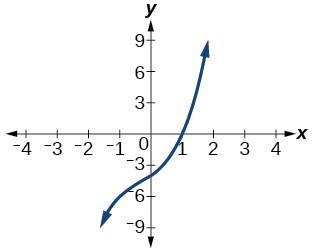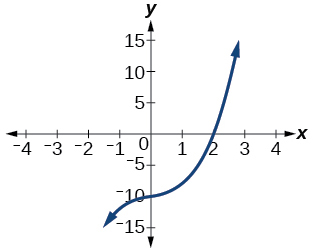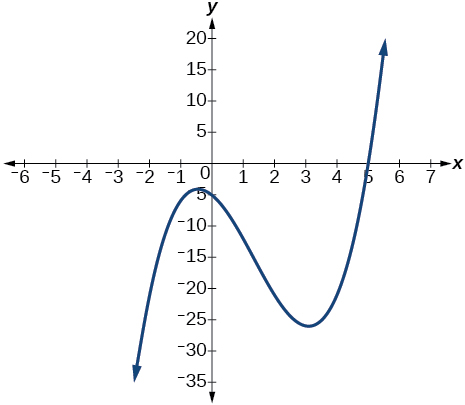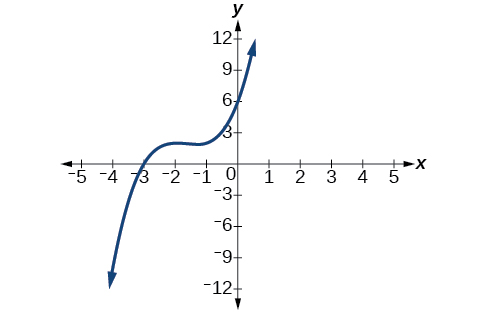# 5.4 Dividing polynomials  (Page 4/6)

 Page 4 / 6

## Verbal

If division of a polynomial by a binomial results in a remainder of zero, what can be conclude?

The binomial is a factor of the polynomial.

If a polynomial of degree $\text{\hspace{0.17em}}n\text{\hspace{0.17em}}$ is divided by a binomial of degree 1, what is the degree of the quotient?

## Algebraic

For the following exercises, use long division to divide. Specify the quotient and the remainder.

$\left({x}^{2}+5x-1\right)÷\left(x-1\right)$

$x+6+\frac{5}{x-1}\text{,}\text{\hspace{0.17em}}\text{quotient:}\text{\hspace{0.17em}}x+6\text{,}\text{\hspace{0.17em}}\text{remainder:}\text{\hspace{0.17em}}\text{5}$

$\left(2{x}^{2}-9x-5\right)÷\left(x-5\right)$

$\left(3{x}^{2}+23x+14\right)÷\left(x+7\right)$

$\left(4{x}^{2}-10x+6\right)÷\left(4x+2\right)$

$\left(6{x}^{2}-25x-25\right)÷\left(6x+5\right)$

$x-5\text{,}\text{\hspace{0.17em}}\text{quotient:}\text{\hspace{0.17em}}x-5\text{,}\text{\hspace{0.17em}}\text{remainder:}\text{\hspace{0.17em}}\text{0}$

$\left(-{x}^{2}-1\right)÷\left(x+1\right)$

$\left(2{x}^{2}-3x+2\right)÷\left(x+2\right)$

$2x-7+\frac{16}{x+2}\text{,}\text{\hspace{0.17em}}\text{quotient:}\text{​}\text{\hspace{0.17em}}2x-7\text{,}\text{\hspace{0.17em}}\text{remainder:}\text{\hspace{0.17em}}\text{16}$

$\left({x}^{3}-126\right)÷\left(x-5\right)$

$\left(3{x}^{2}-5x+4\right)÷\left(3x+1\right)$

$x-2+\frac{6}{3x+1}\text{,}\text{\hspace{0.17em}}\text{quotient:}\text{\hspace{0.17em}}x-2\text{,}\text{\hspace{0.17em}}\text{remainder:}\text{\hspace{0.17em}}\text{6}$

$\left({x}^{3}-3{x}^{2}+5x-6\right)÷\left(x-2\right)$

$\left(2{x}^{3}+3{x}^{2}-4x+15\right)÷\left(x+3\right)$

$2{x}^{2}-3x+5\text{,}\text{\hspace{0.17em}}\text{quotient:}\text{\hspace{0.17em}}2{x}^{2}-3x+5\text{,}\text{\hspace{0.17em}}\text{remainder:}\text{\hspace{0.17em}}\text{0}$

For the following exercises, use synthetic division to find the quotient.

$\left(3{x}^{3}-2{x}^{2}+x-4\right)÷\left(x+3\right)$

$\left(2{x}^{3}-6{x}^{2}-7x+6\right)÷\left(x-4\right)$

$2{x}^{2}+2x+1+\frac{10}{x-4}$

$\left(6{x}^{3}-10{x}^{2}-7x-15\right)÷\left(x+1\right)$

$\left(4{x}^{3}-12{x}^{2}-5x-1\right)÷\left(2x+1\right)$

$2{x}^{2}-7x+1-\frac{2}{2x+1}$

$\left(9{x}^{3}-9{x}^{2}+18x+5\right)÷\left(3x-1\right)$

$\left(3{x}^{3}-2{x}^{2}+x-4\right)÷\left(x+3\right)$

$3{x}^{2}-11x+34-\frac{106}{x+3}$

$\left(-6{x}^{3}+{x}^{2}-4\right)÷\left(2x-3\right)$

$\left(2{x}^{3}+7{x}^{2}-13x-3\right)÷\left(2x-3\right)$

${x}^{2}+5x+1$

$\left(3{x}^{3}-5{x}^{2}+2x+3\right)÷\left(x+2\right)$

$\left(4{x}^{3}-5{x}^{2}+13\right)÷\left(x+4\right)$

$4{x}^{2}-21x+84-\frac{323}{x+4}$

$\left({x}^{3}-3x+2\right)÷\left(x+2\right)$

$\left({x}^{3}-21{x}^{2}+147x-343\right)÷\left(x-7\right)$

${x}^{2}-14x+49$

$\left({x}^{3}-15{x}^{2}+75x-125\right)÷\left(x-5\right)$

$\left(9{x}^{3}-x+2\right)÷\left(3x-1\right)$

$3{x}^{2}+x+\frac{2}{3x-1}$

$\left(6{x}^{3}-{x}^{2}+5x+2\right)÷\left(3x+1\right)$

$\left({x}^{4}+{x}^{3}-3{x}^{2}-2x+1\right)÷\left(x+1\right)$

${x}^{3}-3x+1$

$\left({x}^{4}-3{x}^{2}+1\right)÷\left(x-1\right)$

$\left({x}^{4}+2{x}^{3}-3{x}^{2}+2x+6\right)÷\left(x+3\right)$

${x}^{3}-{x}^{2}+2$

$\left({x}^{4}-10{x}^{3}+37{x}^{2}-60x+36\right)÷\left(x-2\right)$

$\left({x}^{4}-8{x}^{3}+24{x}^{2}-32x+16\right)÷\left(x-2\right)$

${x}^{3}-6{x}^{2}+12x-8$

$\left({x}^{4}+5{x}^{3}-3{x}^{2}-13x+10\right)÷\left(x+5\right)$

$\left({x}^{4}-12{x}^{3}+54{x}^{2}-108x+81\right)÷\left(x-3\right)$

${x}^{3}-9{x}^{2}+27x-27$

$\left(4{x}^{4}-2{x}^{3}-4x+2\right)÷\left(2x-1\right)$

$\left(4{x}^{4}+2{x}^{3}-4{x}^{2}+2x+2\right)÷\left(2x+1\right)$

$2{x}^{3}-2x+2$

For the following exercises, use synthetic division to determine whether the first expression is a factor of the second. If it is, indicate the factorization.

$x-2,\text{\hspace{0.17em}}4{x}^{3}-3{x}^{2}-8x+4$

$x-2,\text{\hspace{0.17em}}3{x}^{4}-6{x}^{3}-5x+10$

Yes $\text{\hspace{0.17em}}\left(x-2\right)\left(3{x}^{3}-5\right)$

$x+3,\text{\hspace{0.17em}}-4{x}^{3}+5{x}^{2}+8$

$x-2,\text{\hspace{0.17em}}4{x}^{4}-15{x}^{2}-4$

Yes $\text{\hspace{0.17em}}\left(x-2\right)\left(4{x}^{3}+8{x}^{2}+x+2\right)$

$x-\frac{1}{2},\text{\hspace{0.17em}}2{x}^{4}-{x}^{3}+2x-1$

$x+\frac{1}{3},\text{\hspace{0.17em}}3{x}^{4}+{x}^{3}-3x+1$

No

## Graphical

For the following exercises, use the graph of the third-degree polynomial and one factor to write the factored form of the polynomial suggested by the graph. The leading coefficient is one.

Factor is $\text{\hspace{0.17em}}{x}^{2}-x+3$Factor is $\text{\hspace{0.17em}}\left({x}^{2}+2x+4\right)$$\left(x-1\right)\left({x}^{2}+2x+4\right)$

Factor is $\text{\hspace{0.17em}}{x}^{2}+2x+5$Factor is $\text{\hspace{0.17em}}{x}^{2}+x+1$$\left(x-5\right)\left({x}^{2}+x+1\right)$

Factor is ${x}^{2}+2x+2$For the following exercises, use synthetic division to find the quotient and remainder.

$\frac{4{x}^{3}-33}{x-2}$

$\text{Quotient:}\text{\hspace{0.17em}}4{x}^{2}+8x+16\text{,}\text{\hspace{0.17em}}\text{remainder:}\text{\hspace{0.17em}}-1$

$\frac{2{x}^{3}+25}{x+3}$

$\frac{3{x}^{3}+2x-5}{x-1}$

$\text{Quotient:}\text{\hspace{0.17em}}3{x}^{2}+3x+5\text{,}\text{\hspace{0.17em}}\text{remainder:}\text{\hspace{0.17em}}0$

$\frac{-4{x}^{3}-{x}^{2}-12}{x+4}$

$\frac{{x}^{4}-22}{x+2}$

$\text{Quotient:}\text{\hspace{0.17em}}{x}^{3}-2{x}^{2}+4x-8\text{,}\text{\hspace{0.17em}}\text{remainder:}\text{\hspace{0.17em}}-6$

## Technology

For the following exercises, use a calculator with CAS to answer the questions.

Consider $\text{\hspace{0.17em}}\frac{{x}^{k}-1}{x-1}\text{\hspace{0.17em}}$ with What do you expect the result to be if $\text{\hspace{0.17em}}k=4?$

Consider $\text{\hspace{0.17em}}\frac{{x}^{k}+1}{x+1}\text{\hspace{0.17em}}$ for What do you expect the result to be if $\text{\hspace{0.17em}}k=7?$

${x}^{6}-{x}^{5}+{x}^{4}-{x}^{3}+{x}^{2}-x+1$

Consider $\text{\hspace{0.17em}}\frac{{x}^{4}-{k}^{4}}{x-k}\text{\hspace{0.17em}}$ for What do you expect the result to be if $\text{\hspace{0.17em}}k=4?$

Consider $\text{\hspace{0.17em}}\frac{{x}^{k}}{x+1}\text{\hspace{0.17em}}$ with What do you expect the result to be if $\text{\hspace{0.17em}}k=4?$

${x}^{3}-{x}^{2}+x-1+\frac{1}{x+1}$

Consider $\text{\hspace{0.17em}}\frac{{x}^{k}}{x-1}\text{\hspace{0.17em}}$ with What do you expect the result to be if $\text{\hspace{0.17em}}k=4?$

## Extensions

For the following exercises, use synthetic division to determine the quotient involving a complex number.

$\frac{x+1}{x-i}$

$1+\frac{1+i}{x-i}$

$\frac{{x}^{2}+1}{x-i}$

$\frac{x+1}{x+i}$

$1+\frac{1-i}{x+i}$

$\frac{{x}^{2}+1}{x+i}$

$\frac{{x}^{3}+1}{x-i}$

${x}^{2}-ix-1+\frac{1-i}{x-i}$

## Real-world applications

For the following exercises, use the given length and area of a rectangle to express the width algebraically.

Length is $\text{\hspace{0.17em}}x+5,\text{\hspace{0.17em}}$ area is $\text{\hspace{0.17em}}2{x}^{2}+9x-5.$

Length is area is $\text{\hspace{0.17em}}4{x}^{3}+10{x}^{2}+6x+15$

$2{x}^{2}+3$

Length is $\text{\hspace{0.17em}}3x–4,\text{\hspace{0.17em}}$ area is $\text{\hspace{0.17em}}6{x}^{4}-8{x}^{3}+9{x}^{2}-9x-4$

For the following exercises, use the given volume of a box and its length and width to express the height of the box algebraically.

Volume is $\text{\hspace{0.17em}}12{x}^{3}+20{x}^{2}-21x-36,\text{\hspace{0.17em}}$ length is $\text{\hspace{0.17em}}2x+3,\text{\hspace{0.17em}}$ width is $\text{\hspace{0.17em}}3x-4.$

$2x+3$

Volume is $\text{\hspace{0.17em}}18{x}^{3}-21{x}^{2}-40x+48,\text{\hspace{0.17em}}$ length is $\text{\hspace{0.17em}}3x–4,\text{\hspace{0.17em}}$ width is $\text{\hspace{0.17em}}3x–4.$

Volume is $\text{\hspace{0.17em}}10{x}^{3}+27{x}^{2}+2x-24,\text{\hspace{0.17em}}$ length is $\text{\hspace{0.17em}}5x–4,\text{\hspace{0.17em}}$ width is $\text{\hspace{0.17em}}2x+3.$

$x+2$

Volume is $\text{\hspace{0.17em}}10{x}^{3}+30{x}^{2}-8x-24,\text{\hspace{0.17em}}$ length is $\text{\hspace{0.17em}}2,\text{\hspace{0.17em}}$ width is $\text{\hspace{0.17em}}x+3.$

For the following exercises, use the given volume and radius of a cylinder to express the height of the cylinder algebraically.

Volume is $\text{\hspace{0.17em}}\pi \left(25{x}^{3}-65{x}^{2}-29x-3\right),\text{\hspace{0.17em}}$ radius is $\text{\hspace{0.17em}}5x+1.$

$x-3$

Volume is $\text{\hspace{0.17em}}\pi \left(4{x}^{3}+12{x}^{2}-15x-50\right),\text{\hspace{0.17em}}$ radius is $\text{\hspace{0.17em}}2x+5.$

Volume is $\text{\hspace{0.17em}}\pi \left(3{x}^{4}+24{x}^{3}+46{x}^{2}-16x-32\right),\text{\hspace{0.17em}}$ radius is $\text{\hspace{0.17em}}x+4.$

$3{x}^{2}-2$

root under 3-root under 2 by 5 y square
The sum of the first n terms of a certain series is 2^n-1, Show that , this series is Geometric and Find the formula of the n^th
cosA\1+sinA=secA-tanA
why two x + seven is equal to nineteen.
The numbers cannot be combined with the x
Othman
2x + 7 =19
humberto
2x +7=19. 2x=19 - 7 2x=12 x=6
Yvonne
because x is 6
SAIDI
what is the best practice that will address the issue on this topic? anyone who can help me. i'm working on my action research.
simplify each radical by removing as many factors as possible (a) √75
how is infinity bidder from undefined?
what is the value of x in 4x-2+3
give the complete question
Shanky
4x=3-2 4x=1 x=1+4 x=5 5x
Olaiya
hi can you give another equation I'd like to solve it
Daniel
what is the value of x in 4x-2+3
Olaiya
if 4x-2+3 = 0 then 4x = 2-3 4x = -1 x = -(1÷4) is the answer.
Jacob
4x-2+3 4x=-3+2 4×=-1 4×/4=-1/4
LUTHO
then x=-1/4
LUTHO
4x-2+3 4x=-3+2 4x=-1 4x÷4=-1÷4 x=-1÷4
LUTHO
A research student is working with a culture of bacteria that doubles in size every twenty minutes. The initial population count was  1350  bacteria. Rounding to five significant digits, write an exponential equation representing this situation. To the nearest whole number, what is the population size after  3  hours?
v=lbh calculate the volume if i.l=5cm, b=2cm ,h=3cm
Need help with math
Peya
can you help me on this topic of Geometry if l help you
litshani
( cosec Q _ cot Q ) whole spuare = 1_cosQ / 1+cosQ
A guy wire for a suspension bridge runs from the ground diagonally to the top of the closest pylon to make a triangle. We can use the Pythagorean Theorem to find the length of guy wire needed. The square of the distance between the wire on the ground and the pylon on the ground is 90,000 feet. The square of the height of the pylon is 160,000 feet. So, the length of the guy wire can be found by evaluating √(90000+160000). What is the length of the guy wire?
the indicated sum of a sequence is known as
how do I attempted a trig number as a starter
cos 18 ____ sin 72 evaluateByByBy OpenStaxBy Dindin SecretoBy Laurence BailenBy Jazzycazz JacksonBy Royalle MooreBy Cath YuBy OpenStaxBy OpenStaxBy Nick SwainBy OpenStax